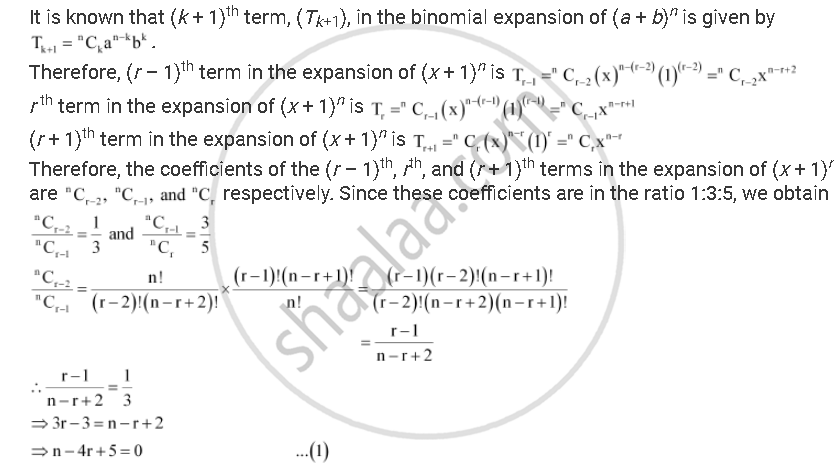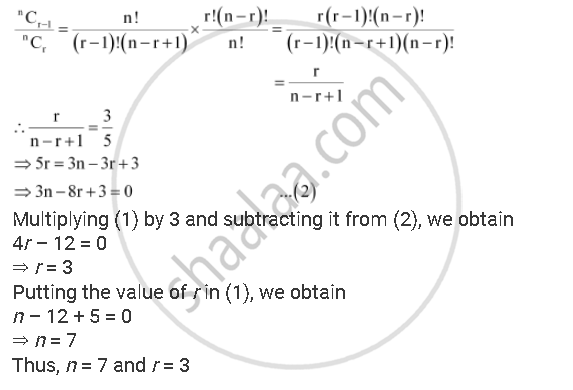Share

# The Coefficients of the (R – 1)Th, Rth and (R + 1)Th Terms in the Expansion of (X + 1)N Are in the Ratio 1:3:5. Find N and R. - Mathematics

#### Question

The coefficients of the (r – 1)thrth and (r + 1)th terms in the expansion of (x + 1)n are in the ratio 1:3:5. Find n and r.

#### SolutionIs there an error in this question or solution?